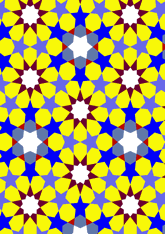# Bourgoin, Plate 108data169/P108

The actual source of this pattern is shown in Qaytbay mosque.

## Geometry

• The symmetry group of the tiling is *632 (p6m).
• All the internal angles of the constituent polygons are a multiple of 1.66 (π/108)°.
• Contains one regular 6-pointed star polygon with vertex angle of 73.33 (11π/27)°.
• Contains one regular 9-pointed star polygon with vertex angle of 80°.
• There are 7 non-regular reflective tiles (including one kite).
• The tiling satisfies the interlace condition and has two finite interlaces and one infinite interlace with straight cross-overs.
• The tiling is edge-to-edge.
• As drawn, contains about 452 polygons.

## References

Publications referenced: